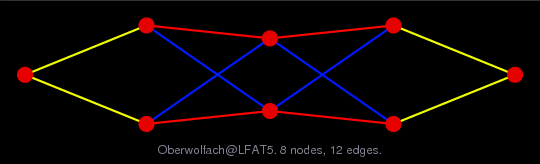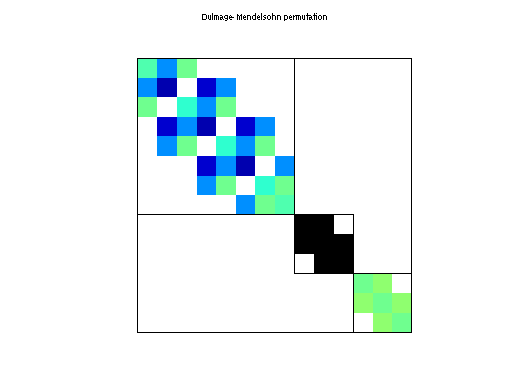Matrix: Oberwolfach/LFAT5

Description: Oberwolfach: linear 1D beam(undirected graph drawing)• Matrix group: Oberwolfach
• download as a MATLAB mat-file, file size: 999 bytes. Use UFget(1440) or UFget('Oberwolfach/LFAT5') in MATLAB.

 Matrix properties number of rows 14 number of columns 14 nonzeros 46 structural full rank? yes structural rank 14 # of blocks from dmperm 3 # strongly connected comp. 3 explicit zero entries 0 nonzero pattern symmetry symmetric numeric value symmetry symmetric type real structure symmetric Cholesky candidate? yes positive definite? yes

 author J. Lienemann, A. Greiner, J. Korvink editor E. Rudnyi date 2004 kind model reduction problem 2D/3D problem? yes

 Additional fields size and type M sparse 14-by-14 E sparse 14-by-14 B sparse 14-by-1 C sparse 1-by-14

Notes:

```Primary matrix in this model reduction problem is the Oberwolfach K matrix
```

 Ordering statistics: result nnz(chol(P*(A+A'+s*I)*P')) with AMD 33 Cholesky flop count 9.1e+01 nnz(L+U), no partial pivoting, with AMD 52 nnz(V) for QR, upper bound nnz(L) for LU, with COLAMD 33 nnz(R) for QR, upper bound nnz(U) for LU, with COLAMD 43

 SVD-based statistics: norm(A) 2.14522e+07 min(svd(A)) 0.149919 cond(A) 1.43092e+08 rank(A) 14 sprank(A)-rank(A) 0 null space dimension 0 full numerical rank? yes

 singular values (MAT file): click here SVD method used: s = svd (full (A)) status: ok